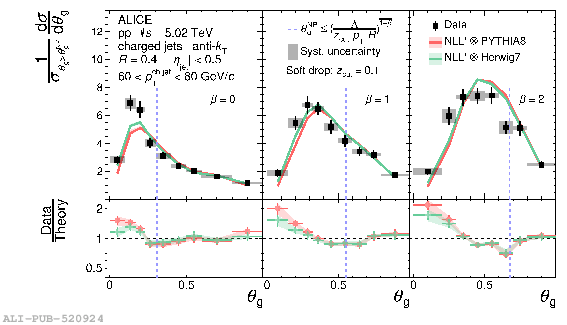# Figure 4

 ALICE measurements of \tg{} distributions in \pp{} collisions at $\sqrt{s}=5.02$ TeV withsoft drop, compared with NLL$^\prime$ predictions carried out with SCET   andcorrected for non-perturbative effects using either PYTHIA8 or Herwig7 . The distributions are normalized such that theintegral of the perturbative region defined by $\tg{} > \tgNP{}$(to the right of the dashed vertical blue line) is unity The non-perturbative scale in Eq. \ref{eq:8} is taken to be $\Lambda=1\;\GeVc$ In determining the normalization, intervals that overlap with the dashed blue line are considered to be in the non-perturbative (left) region.